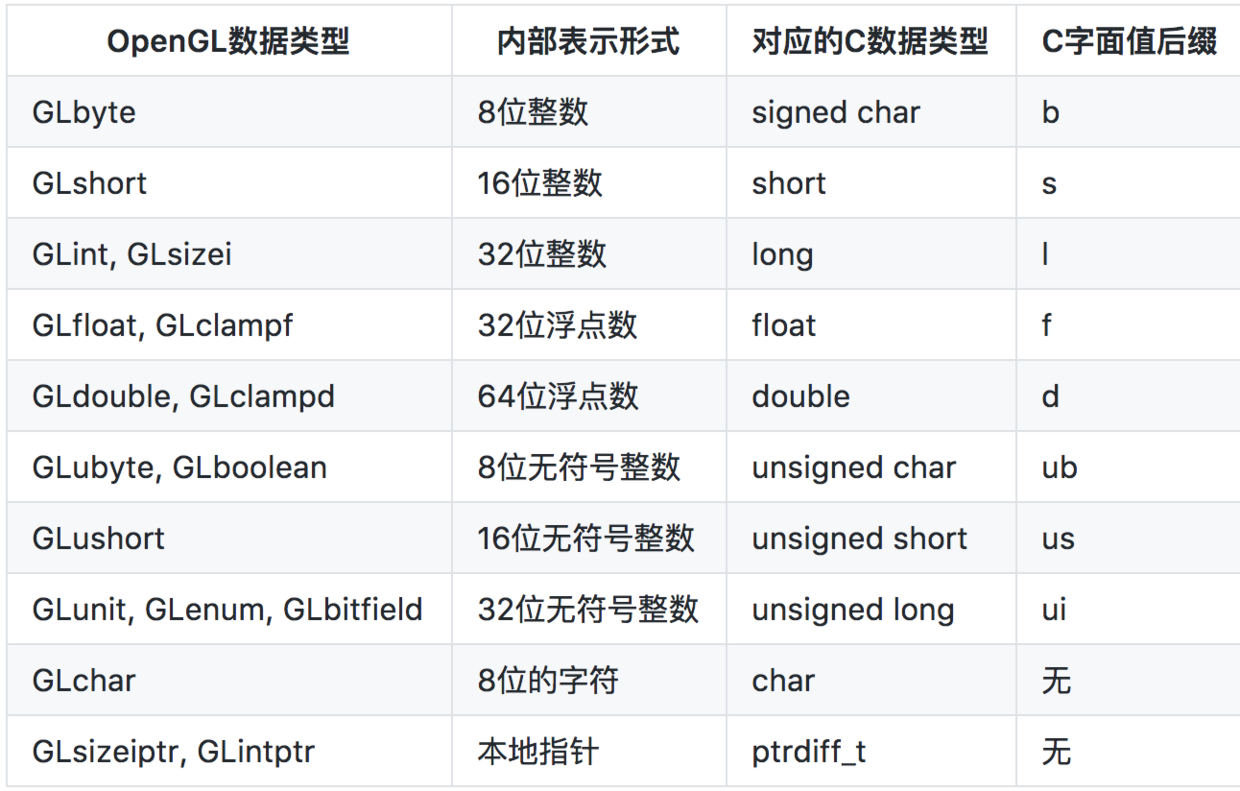# C++中typedef和类型别名

C++ typedef的使用看似十分简单和常用，但是仔细研究一下发现还是有许多需要了解的点，本文主要记录一下学习需要注意的细节。

## 1. 语法和使用场景

typedef是C/C++语言中保留的关键字，用来定义一种数据类型的别名。需要注意的是typedef并没有创建新的类型，只是指定了一个类型的别名而已。typedef定义的类型的作用域只在该语句的作用域之内， 也就是说如果typedef定义在一个函数体内，那么它的作用域就是这个函数。

typedef经常使用的场景包括以下几种：

1. 指定一个简单的别名，避免了书写过长的类型名称
2. 实现一种定长的类型，在跨平台编程的时候尤其重要
3. 使用一种方便阅读的单词来作为别名，方便阅读代码

#### 1.1 减少书写类型的长度

///第一种方式
struct MyStruct {
int data1;
char data2;
};
//之后定义变量
struct MyStruct a, b;

///第二种方式（声明的同时定义）
struct MyStruct {
int data1;
char data2;
}a, b;

///第三种方式（不需要提供结构体名字，直接定义）
struct {
int data1;
char data2;
}a, b;

typedef struct _RECT {
LONG left;
LONG top;
LONG right;
LONG bottom;
} RECT, *PRECT;

///第一种情况
struct MyStruct {
int data1;
char data2;
};
typedef struct MyStruct newtype;
newtype a, b;

///第二种情况
typedef struct MyStruct {
int data1;
char data2;
} newtype;
newtype a, b;

///第三种情况
typedef struct {
int data1;
char data2;
} newtype;
newtype a, b;

#### 1.2 实现一种定长的类型#### 1.3 实现一种有意义可读的别名

int current_speed ;
int high_score ;

void congratulate(int your_score) {
if (your_score > high_score)
...
}

typedef int km_per_hour ;
typedef int points ;

km_per_hour current_speed ;
points high_score ;

void congratulate(points your_score) {
if (your_score > high_score)
...
}

    unsigned int a;         // Okay
unsigned km_per_hour b; // 编译报错
long int c;             // Okay
long km_per_hour d;     // 编译报错

## 2. 其他情况下typedef的使用

typedef在定义复杂类型时，有时候会变得难于理解，有很多时候这些使用方式看起来并没有什么严格上的逻辑可言，需要使用者自己熟悉。

### 2.1 定义指针

        //语法： typedef  指针类型  别名
typedef  char*  CHARS；

1. 指针自己是常量，指针不能被修改（在C++11种被称为 Top-Level const）
2. 指针指向的变量是常量，指针指向的变量不能修改（在C++11种被称为Low-Level const）

    const double pi = 3.1415;
double p = 3.0;

const double * ptoConst = &pi;   //指向const double的指针（ptoConst可以指向其他const变量）
double * const constp = &p;      //指向double类型的常量指针（可以解引用修改p的值）
const double * const constptoconst = &pi; //指向const double类型的const指针

typedef char* CHARS;
typedef CHARS const CPTR_1;
typedef char* const CPTR_2;
typedef const CHARS CPTR_3;
typedef const char* CPTR_4;

1. CHARS == char*
2. CPTR_1 == char* const [Top-Level const]
3. CPTR_2 == char* const [Top-Level const]
4. CPTR_3 == char* const [Top-Level const]
5. CPTR_4 == char const * (const char *) [Low-Level const]

1. typedef 并不是#define，不能简单的替换字符来理解
2. typedef 将中间变量类型作为一个整体来看，也就是：typederf xxx 别名，这里面xxx如果包含修饰符，那么修饰符要整体看待，也就是整个xxx才是一种类型。（以CPTR_3为例，CHARS是指针类型，那么给它加上const，也就是整体xxx是 const类型的指针，于是CPTR_3的释义是：const类型的char*指针（指针是const类型的：Top-Level const）

C++ templates一书中提倡将 const修饰的变量写在 const 所修饰的变量前面，也就是：

//const的变量是int类型的
const int i = 10;
int const i = 10; //(推荐写法）

//const的变量是int*类型的
int* const pi = &i;//（int*是const的，也就是说是指针是const，Top-Level const）

//指向const int的指针 [Low-Level const]
int const *pi = &i;//（推荐写法）
const int *pi = &i; 

### 2.2 定义数组

typedef char arrType;  //arrType是一个包含6个参数的数组类型

int s；而不是 int s;

### 2.3 定义函数指针

typedef在C/C++中还有一个非常常见的使用场景是定义函数指针，语法如下：

typedef int (*MathFunc)(float, int);

### 2.4 更加复杂的定义

理解复杂声明可用的“右左法则”：从变量名看起，先往右，再往左，碰到一个圆括号就调转阅读的方向；括号内分析完就跳出括号，还是按先右后左的顺序，如此循环，直到整个声明分析完。举例：
int (*func)(int *p);

int (*func)(int *);
func右边是一个[]运算符，说明func是具有5个元素的数组；func的左边有一个*，说明func的元素是指针（注意这里的*不是修饰func，而是修饰func的，原因是[]运算符优先级比*高，func先跟[]结合）。跳出这个括号，看右边，又遇到圆括号，说明func数组的元素是函数类型的指针，它指向的函数具有int*类型的形参，返回值类型为int。

## 3. C++11下新的选择（使用using定义别名）

C++11 中扩展了using的使用场景（C++11之前using主要用来引入命名空间名字 如：using namespace std；），可以使用using定义类型的别名：

using 别名 = xxx(类型）;

    typedef int points;
using points = int; //等价的写法

typedef void (*FP) (int, const std::string&);
using FP = void (*) (int, const std::string&); //等价的using别名

template <typename T>
using Vec = MyVector<T, MyAlloc<T>>;

// usage
Vec<int> vec;

template <typename T>
typedef MyVector<T, MyAlloc<T>> Vec;

// usage
Vec<int> vec;

template <typename T>
struct Vec
{
typedef MyVector<T, MyAlloc<T>> type;
};

// usage
Vec<int>::type vec;

template <typename T>
class Widget
{
typename Vec<T>::type vec;
};

template <typename T>
class Widget
{
Vec<T> vec;
};

A typedef-name can also be introduced by an alias-declaration. The identifier following the using keyword becomes a typedef-name and the optional attribute-specifier-seq following the identifier appertains to that typedef-name. It has the same semantics as if it were introduced by the typedef specifier. In particular, it does not define a new type and it shall not appear in the type-id.

### 参考文献

1. Wikipedia typedef
2. 结构体
3. typedef和const之间的trap
4. C++ typedef interpretation of const pointers
5. [C++ templates: The Complete Guide –1.4 Some Remarks About Programming Style
]
6. 关于typedef的用法总结
7. 蓝色的味道知乎：Effective Modern C++ Note 02

©️2019 CSDN 皮肤主题: 大白 设计师: CSDN官方博客